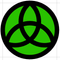cancel
Showing results for
Did you mean:
cancel
Showing results for
Did you mean:
Developer forum

## Lock or constraint A/B defaults?Hi,
LOCK ALL under paramters lock everything.
Say I want to lock only A size or constrint A/B size. Whaddoido?AC 25 SWE Full

HP Zbook Fury 15,6 G8. 32 GB RAM. Nvidia RTX A3000.
1 ACCEPTED SOLUTION
6 REPLIES 6Champion
Does Lock "Parameter Name" not work for you?

http://gdl.graphisoft.com/reference-guide/the-parameter-script#LOCK_gdlcommand

You can also Hide the parameters so users can't enter different values.
Apple iMac macOS Ventura / AC26UKI (most recent builds)DGSketcher wrote:
Does Lock "Parameter Name" not work for you?

http://gdl.graphisoft.com/reference-guide/the-parameter-script#LOCK_gdlcommand

You can also Hide the parameters so users can't enter different values.
Haha...I'm so daft. I figured the quotation marks was just for "put your name here" since ALL was written without quotation marks. Then all isa fine but locking the A/B ratio...i e I want to be able to resize but not distort.
AC 25 SWE Full

HP Zbook Fury 15,6 G8. 32 GB RAM. Nvidia RTX A3000.
SolutionExpert
Hi Mats,

Is one of the values (a, b) the determining factor or do you want them both to be editable. If so do this:
```
parameters b = a*ratio
```
if you want them both to be determining factors then you need to use the glob_modpar_name to determine which parameter is being edited, like this:
lets first assume the ratio of a:b is 5:3 therefore
aRatio = 5/3
bRatio = 3/5 or 1/aRatio
```
if glob_modpar_name = "a" then
parameters b = a*(1/aRatio)
endif
if glob_modpar_name = "b" then
parameters a = b*aRatio
endif
```
Actually, to make sure the response is instantaneous you should establish the value in a local value before sending it to the actual parameter. So the script should read:
```
if glob_modpar_name = "a" then
b = a*(1/aRatio)
parameters b = b
endif
if glob_modpar_name = "b" then
a = b*aRatio
parameters a = a
endif
```
Coding like this will make the object respond without having to refresh the parameters.
Creator of Cadswift's parametric GDL libraries
Creator of Infinite Openings and Component Catalogues
Push the envelope & watch it bendKristian wrote:
Hi Mats,

Is one of the values (a, b) the determining factor or do you want them both to be editable. If so do this:
```
parameters b = a*ratio
```
if you want them both to be determining factors then you need to use the glob_modpar_name to determine which parameter is being edited, like this:
lets first assume the ratio of a:b is 5:3 therefore
aRatio = 5/3
bRatio = 3/5 or 1/aRatio
```
if glob_modpar_name = "a" then
parameters b = a*(1/aRatio)
endif
if glob_modpar_name = "b" then
parameters a = b*aRatio
endif
```
Actually, to make sure the response is instantaneous you should establish the value in a local value before sending it to the actual parameter. So the script should read:
```
if glob_modpar_name = "a" then
b = a*(1/aRatio)
parameters b = b
endif
if glob_modpar_name = "b" then
a = b*aRatio
parameters a = a
endif
```
Coding like this will make the object respond without having to refresh the parameters.
Hi Kristian and thanks! I'll put that in my code. I should buy some hours of GDL-training from you. I dabble with softer stuff like a 2D (yeah...) like: a 2D-tree symbol made only to look nice. I'm working on a 3D-tree and I will try to implement this 2D together with renovation filter control as well...
AC 25 SWE Full

HP Zbook Fury 15,6 G8. 32 GB RAM. Nvidia RTX A3000.Expert
That is a really nice looking 2D tree symbol.
I'd be more than happy to give you some GDL training if there are specific things you want to learn. However, if you just have simple questions, like this topic, I am always happy to answer them free of charge.
Creator of Cadswift's parametric GDL libraries
Creator of Infinite Openings and Component Catalogues
Push the envelope & watch it bendKristian wrote:
That is a really nice looking 2D tree symbol.
I'd be more than happy to give you some GDL training if there are specific things you want to learn. However, if you just have simple questions, like this topic, I am always happy to answer them free of charge.AC 25 SWE Full

HP Zbook Fury 15,6 G8. 32 GB RAM. Nvidia RTX A3000.Latest solutions

Most liked posts